Example Questions

Example Question #51 : Linear / Rational / Variable Equations

Jon invested part of $16,000 at 3% and the rest at 5% for a total return of$680.

Quantity A: The amount Jon invested at 5% interest

Quantity B: The amount Jon invested at 3% interest

Quantity A is greater

Quantity B is greater

The relationship cannot be determined from the information given

The two quantities are equal

Quantity A is greater

Explanation:

First, letrepresent the invested amount at 3% and set up an equation like this: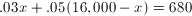Solve for, and you'll find that Jon invested $6,000 at 3% and$10,000 at 5%.

Example Question #52 : Equations / Inequalities

Audrey, Penelope and Clementine are all sisters. Penelope is 8 years older than Clementine and 2 years younger than Audrey. If the sum of Penelope and Clementine's age is Audrey's age, how old is Clementine's age?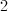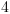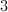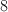Explanation:

Let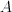= Audrey's age,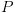= Penelope's age, and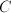= Clementine's age.

Since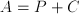, then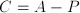.

Furthermore,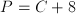, and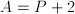.

Through substitution,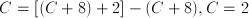.

Example Question #1851 : Sat Mathematics

If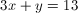and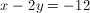, what is the value of?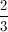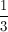Explanation:

We could use the substitution or elimination method to solve the system of equations. Here we will use the elimination method.

To solve for, combine the equations in a way that makes the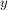terms drop out. The first equation hasand the second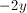, so multiplying the first equation times 2 then adding the equations will eliminate theterms.

Multiplying the first equation times 2: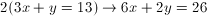Adding this result to the second equation: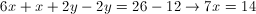Isolateby dividing both sides by 7: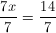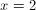Example Question #71 : Linear / Rational / Variable Equations

If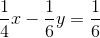and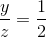, then what is the value of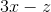?Explanation:

Since the expression we want just involves z and x, but not y, we start by solvingfor y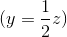.

Then we can plug that expression in for y in the first equation.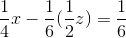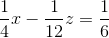Multiply everything by 12 to get rid of fractions.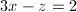Example Question #55 : Equations / Inequalities

If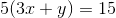, what isin terms of?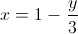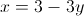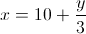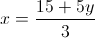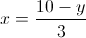Explanation:

Use inverse operations to isolate x. Working from the outermost part on the left side, we first divide both sides by 5.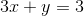To isolate the x term, subtract y from both sides.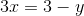Finally, isolating just x, divide both sides by 3.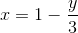Example Question #82 : Equations / Inequalities

If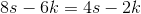, then, in terms of,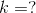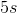Cannot be determined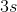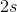Explanation:

You can solve this problem by plugging in random values or by simply solving for k. To solve for k, put the s values on one side and the k values on the other side of the equation. First, subtract 4s from both sides. This gives 4s – 6k = –2k. Next, add 6k to both sides. This leaves you with 4s = 4k, which simplifies to s=k. The answer is therefore s.

Example Question #91 : Algebra

The sum of two consecutive odd integers is 32. What is the value of the next consecutive odd integer?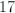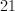Cannot be determined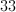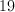Explanation:

Let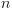be the smallest of the two consecutive odd integers. Thus,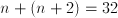and it follows that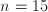.

We have that 15 and 17 are the consecutive odd integers whose sum is 32, so the next odd integer is 19.

Example Question #82 : Linear / Rational / Variable Equations

John has $50 for soda and he must buy both diet and regular sodas. His total order must have at exactly two times as many cans of diet soda as cans of regular soda. What is the greatest number of cans of diet soda John can buy if regular soda is$0.50 per can and diet soda is \$0.75 per can?

51

25

50

75

50

Explanation:

From our data, we can come up with the following two equations:

0.50R + 0.75D = 50

2R = D

Replace the D value in the second equation into the first one:

0.5R + 0.75 * 2R = 50

0.5R + 1.5R = 50; 2R = 50; R = 25

However, note that the question asks for the number of diet cans, so this will have to be doubled to 50.

Example Question #51 : Linear / Rational / Variable Equations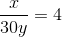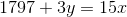Quantity AQuantity BThe relationship cannot be determined from the information given.

Quantity B is greater.

Quantity A is greater.

The quantities are equal.

The quantities are equal.

Explanation:

In order to solve for y, place x in terms of y in the first equation and then substitute that for x in the second equation.

The first equation would yield: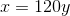Substituting into the second equation, we get: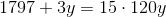Simplify: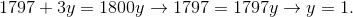Example Question #1 : Algebra

If 6x = 42 and xk = 2, what is the value of k?

7

2/7

1/7

5

1/6